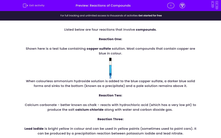# Finding the HCF of Algebraic Expressions

In this worksheet, students find the highest common factor of two algebraic expressions.Key stage:  KS 3

Curriculum topic:   Algebra

Curriculum subtopic:   Understand Expressions, Equations, Inequalities, Terms and Factors

Difficulty level:#### Worksheet Overview

This worksheet is about finding the Highest Common Factor (HCF) of two algebraic expressions.

Example 1

Find the HCF of 3p2qr3 and 6pq3r2

Take each term in turn.

HCF of 3 and 6 is 3.
HCF of p2 and p is p.
HCF of q and q3 is q.
HCF of r3 and r2 is r2.

So, required HCF is 3pqr2.

Example 2

Find the HCF of 4y(y - 7)(7y + 4) and (y - 7)2(7y + 4)

The highest terms common to both are (y - 7) and (7y + 4).

So the HCF is (y - 7)(7y + 4)

### What is EdPlace?

We're your National Curriculum aligned online education content provider helping each child succeed in English, maths and science from year 1 to GCSE. With an EdPlace account you’ll be able to track and measure progress, helping each child achieve their best. We build confidence and attainment by personalising each child’s learning at a level that suits them.

Get started